1. Five resistors of equal resistance are connected to a cell as shown.What is correct about the power dissipated in the resistors?

2. The electromotive force (emf) of a cell is defined as
3. A current is established in a coil of wire in the direction shown.The direction of the magnetic field at point P is

4. A cell with negligible internal resistance is connected as shown. The ammeter and the voltmeter are both ideal.What changes occur in the ammeter reading and in the voltmeter reading when the resistance of the variable resistor is increased?5. Which diagram best represents the electric field due to a negatively charged conducting sphere?
6. The circuit shows a resistor R connected in series with a battery and a resistor of resistance 10 Ω. The emf of the battery is 20 V and it has negligible internal resistance. The current in the circuit is 1.0 A.Which of the following is the resistance of R?

7. Three identical resistors are connected to a battery as shown.Which of the following is a correct statement?

8. The diagram shows two current-carrying wires, P and Q, that both lie in the plane of the paper. The arrows show the conventional current direction in the wires.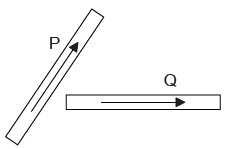The electromagnetic force on Q is in the same plane as that of the wires. What is the direction of the electromagnetic force acting on Q?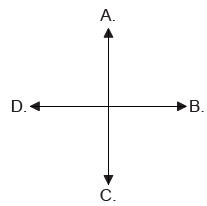9. A positively-charged particle moves parallel to a wire that carries a current upwards.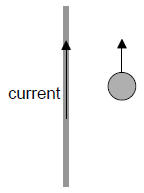What is the direction of the magnetic force on the particle?

10. An electron travelling at speed v perpendicular to a magnetic field of strength B experiences a force F.

What is the force acting on an alpha particle travelling at 2v parallel to a magnetic field of strength 2B?

11. An electron is accelerated through a potential difference of 2.5 MV. What is the change in kinetic energy of the electron?
12. Which of the following diagrams illustrates the electric field pattern of a negatively charged sphere?
13. In the circuits below the cells have the same emf and zero internal resistance. The resistors all have the same resistance.Which of the following gives the ratio power dissipated in X14. A positively charged particle enters the space between two charged conducting plates, with a constant velocity directed parallel to the plates, as shown.The top plate is positively charged and the bottom plate is negatively charged. There is a magnetic field in the shaded region PQRS. The particle continues to move in a horizontal straight line between the plates. Which of the following correctly describes the magnetic field direction?

15. A beam of electrons moves between the poles of a magnet.What is the direction in which the electrons will be deflected?

16. A cell has an emf of 4.0 V and an internal resistance of 2.0 Ω. The ideal voltmeter reads 3.2 V.What is the resistance of R?

17. Three resistors are connected as shown. What is the value of the total resistance between X and Y?18. Two resistors X and Y are made of uniform cylinders of the same material. X and Y are connected in series. X and Y are of equal length and the diameter of Y is twice the diameter of X.The resistance of Y is R.

What is the resistance of this series combination?

19. A metal wire X with length L and radius r has a resistance R. A wire Y of length 4L made from the same material as X has the same resistance R. What is the radius of Y?
20. A cylindrical resistor of length

l is made from a metal of mass m. It has a resistance R.

Two resistors, each of length 2l and mass m/2, are then created from the same volume of the metal.

What is the resistance of the two resistors when connected in parallel?

21. Two rectangular blocks, X and Y, of the same material have different dimensions but the same overall resistance. Which of the following equations is correct?
22. A cell of emf ε and internal resistance r delivers current to a small electric motor.450 C of charge flows through the motor and 9000 J of energy are converted in the motor. 1800 J are dissipated in the cell. The emf of the cell is

23. A resistor of resistance 12 Ω is connected in series with a cell of negligible internal resistance. The power dissipated in the resistor is P. The resistor is replaced with a resistor of resistance 3.0 Ω. What is the power dissipated in this resistor?
24. A proton is accelerated from rest through a potential difference of 1000 V. What is the potential difference through which an alpha particle must be accelerated to gain the same kinetic energy as the accelerated proton?
25. In the circuit shown, the fixed resistor has a value of 3 Ω and the variable resistor can be varied between 0 Ω and 9 Ω.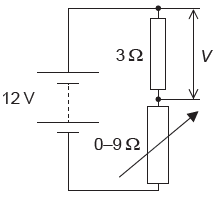The power supply has an emf of 12 V and negligible internal resistance. What is the difference between the maximum and minimum values of voltage V across the 3 Ω resistor?

26. The diagram shows two equal and opposite charges that are fixed in place.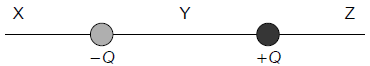At which points is the net electric field directed to the right?

27. A wire carrying a current

I is at right angles to a uniform magnetic field of strength B. A magnetic force F is exerted on the wire. Which force acts when the same wire is placed at right angles to a uniform magnetic field of strength 2B when the current is I/4?

28. A –5µC charge and a +10µC charge are a fixed distance apart.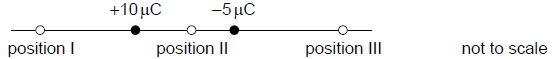Where can the electric field be zero?

29. Three positive point charges of equal magnitude are held at the corners X, Y and Z of a right-angled triangle. The point P is at the midpoint of XY. Which of the arrows shows the direction of the electric field at point P?30. An electron enters the vacuum between two oppositely charged plates with velocity

v. The electron is followed by an alpha particle moving with the same initial velocity as the electron. A uniform magnetic field is directed out of the plane of the paper.The electron’s path is undeflected. The path of the alpha particle will be

31. A liquid that contains negative charge carriers is flowing through a square pipe with sides A, B, C and D. A magnetic field acts in the direction shown across the pipe.

On which side of the pipe does negative charge accumulate?32. An electron enters the region between two charged parallel plates initially moving parallel to the plates.The electromagnetic force acting on the electron

33. Two resistors, made of the same material, are connected in series to a battery. The length of resistor X is twice that of resistor Y, and X has twice the cross-sectional area of Y.Which of the following gives resistance of X34. A +3 C charge and a −4 C charge are a distance x apart. P is a distance x from the +3 C charge on the straight line joining the charges.What is the magnitude of the electric field strength at P?

35. What is the definition of electric current?
36. The magnetic field produced by a current in a straight wire is in
37. Which of the following will not give rise to a magnetic field?
38. One electronvolt is equal to
39. An electron passes the north pole of a bar magnet as shown below.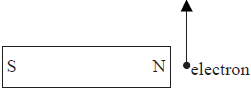What is the direction of the magnetic force on the electron?

40. Two electrodes, separated by a distance d, in a vacuum are maintained at a constant potential difference. An electron, accelerated from one electrode to the other, gains kinetic

energy Ek.

The distance between the electrodes is now changed to 1/3d.

What is the gain in kinetic energy of an electron that is accelerated from one electrode to the other?

41. A battery of internal resistance 2 Ω is connected to an external resistance of 10 Ω. The current is 0.5 A.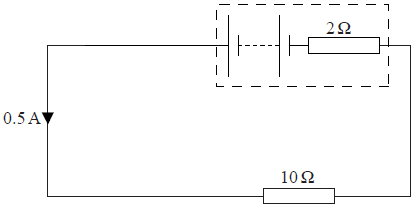What is the emf of the battery?

42. A battery of emf 6.0V is connected to a 2.0Ω resistor. The current in the circuit is 2.0A. The internal resistance of the battery is
43. Coulomb’s law refers to electric charges that are
44. An electric circuit consists of three identical resistors of resistance R connected to a cell of emf ε and negligible internal resistance.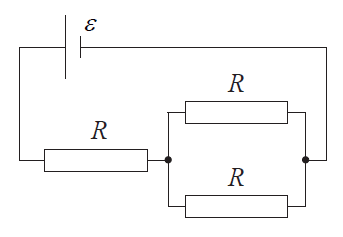What is the magnitude of the current in the cell?

45. Three resistors of resistance R are connected in parallel across a cell of electromotive force (emf) V that has a negligible internal resistance. What is the rate at which the cell supplies energy?